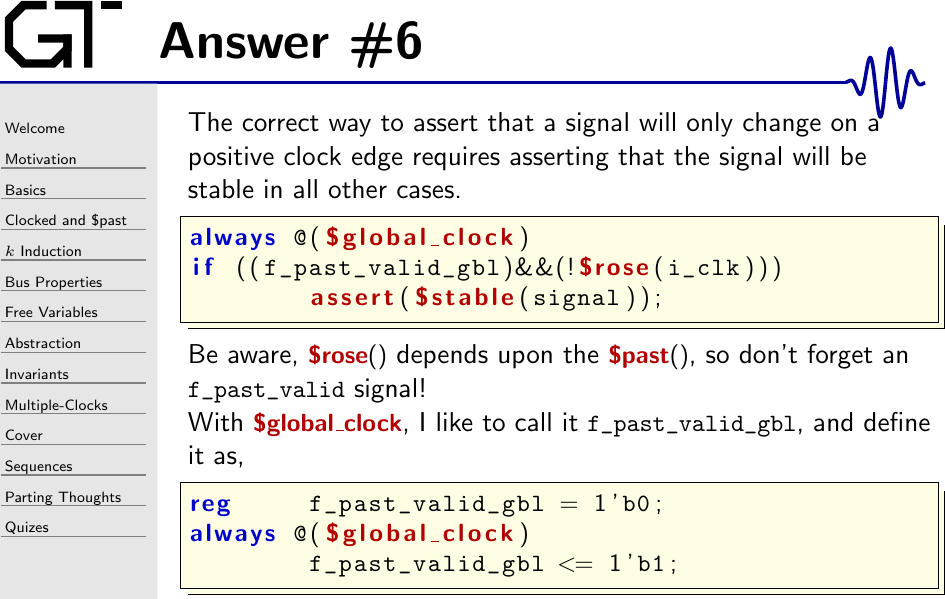The correct assertion basically says that if the clock didn’t rise, then the signal shouldn’t change either. Further, the correct assertion must avoid referencing any `\$past()` values within any assertions on the initial timestep. Therefore, while the `!\$rose(i_clk)` example comes the closest, even this isn’t quite right.

Let’s walk through these choices.

The `\$fell(i_clk)` assertion doesn’t capture what we want. You can see this in Fig. 1 below.Here, you can see that `\$fell(i_clk)` is only ever true if the clock falls. If ever the clock takes more than two formal timesteps, the `signal` will be unconstrained as shown in Fig. 1 above. While unconstrained, the solver is free to make it take on any value.

The `!\$past(i_clk))` assertion is also similarly wrong. This is shown in Fig. 2 below.Here you can see that `!\$past(i_clk)` only constrains our signal over half the clock period, not the whole clock period. The second, and worse problem, is that on the one clock period when we do want to allow `signal` to change, `!\$past(i_clk)` is true. A third more subtle problem is that `!\$past(i_clk)` isn’t constrained on the initial timestep, and neither is the `\$past(signal)` function upon with `\$stable(signal)` depends. As a result, our signal is both over and underconstrained and far from what we want, and guaranteed to fail any assertion.

But let’s take a closer look at Fig. 2 for a moment. You’ll then notice that the one and only clock period we want to allow a change from the previous period is when the expression, `i_clk && !\$past(i_clk)`, is true. This expression is equivalent to `\$rose(i_clk)`. Therefore we should be able to insist that `signal` be stable all other times or, equivalently, whenever `!\$rose(i_clk)`.

Now we get to the subtle problem. That is, whether you are using `\$rose(i_clk)` or `\$fell(i_clk)`, both internally depend upon `\$past(i_clk)`–something that is undefined on the first timestep. There are two ways around this.

Using the Symbiotic EDA Suite, this could be eaxpressed simply with,

In this case, the `1 ##1` will cause the solver to skip the first timestep, and then perform the check on the second time step where `\$rose()`, `\$stable()`, etc. will be valid.

The other way around this would be to use an `f_past_valid` construct again.

Using these assertions, you should be able to achieve a waveform similar to the one shown in Fig. 3 below.Do be careful when doing this, however, since the `f_past_valid` that’s appropriate for `gbl_clk` isn’t the same as the `f_past_valid` that would be appropriate for `i_clk`. This is why I labeled the `f_past_valid` used in the slide above as `f_past_valid_gbl`, to indicate that it’s an `f_past_valid` type of signal, but specifically defined for the `gbl_clk` timestep.